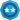Choose a Product Community
Resources

## Sum the length of variuos FindAll searches

SOLVEDContributor

## Sum the length of variuos FindAll searches

Hi to everyone, I have to count the total number of elements present in a web page (see image). The total number is 7 and the only way to find them is to do FindAll 3 times, one for every name of the target (DR 4010 PT100, TelevisIn and TelevisGo). After the FindAll I put it in an Array and then I get the length of the Array using .length (see below)

var Instrument = new Array("DR 4010 PT100", "TelevisIn", "TelevisGo")
for (var i=0; i<Instrument.length;i++)
{
var Instrument_Count = Equipment_Targets_List.FindAll("contentText", Instrument[i], 15, true).toArray()
var Instrument_Sum = Instrument_Count.length

}

How can I sum the 3 length values?

2 REPLIES 2Champion Level 2

If I understand correctly, you want the sum to be 7, as you have 7 boxes? It will be something like,

``````var Instrument_Sum = 0
var Instrument = new Array("DR 4010 PT100", "TelevisIn", "TelevisGo")
for (var i = 0; i < Instrument.length; i++) {
var Instrument_Count = Equipment_Targets_List.FindAll("contentText", Instrument[i], 15, true).toArray()
Instrument_Sum += Instrument_Count.length
}``````Contributor

Thank you very much!cancel
Showing results for